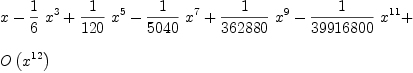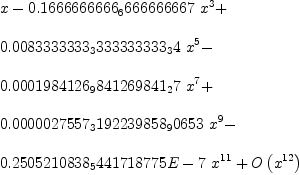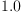login  home  contents  what's new  discussion  bug reports help  links  subscribe  changes  refresh  edit

 Submitted by : (unknown) at: 2007-11-17T21:54:40-08:00 (16 years ago) Name : Axiom Version : default friCAS-20090114 Axiom-20050901 OpenAxiom-20091012 OpenAxiom-20110220 OpenAxiom-Release-141 Category : Axiom Aldor Interface Axiom Compiler Axiom Library Axiom Interpreter Axiom Documentation Axiom User Interface building Axiom from source lisp system MathAction Doyen CD Reduce Axiom on Windows Axiom on Linux Severity : critical serious normal minor wishlist Status : open closed rejected not reproducible fix proposed fixed somewhere duplicate need more info Optional subject :   Optional comment :

It seems that the interpreter handles strangely the power series:

fricas
a:= series sin(x)(1)
Type: UnivariatePuiseuxSeries?(Expression(Integer),x,0)
fricas
)di type a
Type of value of a: Any
-- If I coerce it...
a:=a::UnivariatePuiseuxSeries(Expression Float,x,0)(2)
Type: UnivariatePuiseuxSeries?(Expression(Float),x,0.0)
fricas
)di type a
Type of value of a: UnivariatePuiseuxSeries(Expression(Float),x,0.0)
a*1.0(3)
Type: UnivariatePuiseuxSeries?(Expression(Float),x,0.0)

Fatal bug:
 a:= series sin(x);
a*1.0;
>> System error:
Caught fatal error [memory may be damaged]
protected-symbol-warn called with (NIL)


From trace it seems that the interpreter doesn't coerce one args in Expression (Float):

  1<enter valueArgsEqual? : (|UnivariatePuiseuxSeries| (|Expression| (|Integer|)) |x|
((0 . 0) 0 . 1))\
(|UnivariatePuiseuxSeries| (|Expression| (|Float|)) |x| ((0 . 0) 0 . 1))
...


And here:

 1<enter algEqual : ((0 . 0) 0 . 1)\((0 0 . 0) 0 1 . 0)\(|Expression| (|Float|))
1<enter compiledLookupCheck : =\((|Boolean|) $!$)\#<vector 08eccc78>
1>exit compiledLookupCheck : (#<compiled-function |EXPR;=;2\$B;21|> . #<vector 08eccc78>)


after SPADCALL (it's not traced) "=" in EXPR trigger the bug:

  algEqual : ((0 . 0) 0 . 1)\((0 0 . 0) 0 1 . 0)\(|Expression| (|Float|))
^^^^^^^^^^^^^^^


I think above is not of type EXPR FLOAT.

Other thing:

fricas
1.0::UnivariatePuiseuxSeries(Expression Float,x,0.0)(4)
Type: UnivariatePuiseuxSeries?(Expression(Float),x,0.0)

Memory damaged if it's added to .axiom.input

#132
Series expansion with complex doesn't print expansion point in type expression
#136
Coercion of power series

Transferred from savannah --unknown, Thu, 31 Mar 2005 11:51:06 -0600 reply
Idem

bug #10063 (project axiom)

Some information --unknown, Wed, 01 Jun 2005 13:17:08 -0500 reply